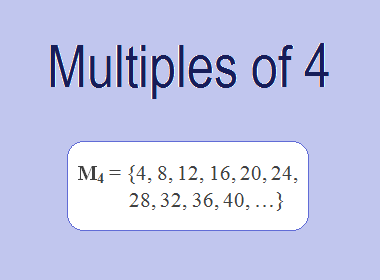# Multiples of 4The Multiples of 4 are 4, 8, 12, 16, 20, 24, 28, 32, 36, 40, … i.e. M4 = {4, 8, 12, 16, 20, 24, 28, 32, 36, 40, …}. Multiples of 4 are the series of numbers obtained by multiplying 4 with the natural numbers.

4 × 1 = 4

4 × 2 = 8

4 × 3 = 12

4 × 4 = 16

4 × 5 = 20

4 × 6 = 24

4 × 7 = 28

4 × 8 = 32

4 × 9 = 36

4 × 10 = 40

And so on.

From this series, we observe that the multiples of 4 can be derived by multiplying 4 by natural numbers in ascending order. These multiples form an arithmetic sequence with a common difference of 4.

## Properties of Multiples of 4

Multiples of 12 possess several interesting properties that make them unique. Here are a few notable ones:

1.    Divisibility by 4: Every multiple of 4 is divisible by 4 without leaving a remainder. This property applies universally to all multiples of 4, as they can be expressed as 4 multiplied by an integer.

2.    Even Numbers: Multiples of 4 are always even numbers. An even number is any integer that is divisible by 2. Since 4 is divisible by 2, all its multiples will also be divisible by 2, making them even.

3.    Increasing by 4: Each subsequent multiple of 4 is obtained by adding 4 to the previous multiple. For instance, starting from 4, we add 4 to get 8, then 12, and so on. This pattern continues indefinitely.

********************

********************

## Patterns and Observations:

1.    Digit Sum: If we observe the digit sums of multiples of 4, we notice a pattern. The digit sums alternate between 4 and 8. For example, the digit sum of 4 is 4, while the digit sum of 8 is 8. Similarly, the digit sum of 12 is 3 (1 + 2 = 3), and the digit sum of 16 is 7 (1 + 6 = 7). This pattern of alternating digit sums repeats indefinitely.

2.    Repeating Last Two Digits: Another interesting pattern emerges when we look at the last two digits of multiples of 4. The last two digits of the multiples follow a cyclical pattern: 04, 08, 12, 16, 20, 24, and so on. This cyclic nature is a result of the fact that each multiple is formed by adding 4 to the previous multiple.

## Applications of Multiples of 4

The study of multiples of 4 finds applications in various fields, including mathematics, science, and everyday life:

1.    LCMOne of the applications of multiples of 4 is in finding the lowest common multiple (LCM) of two or more numbers. The LCM is the lowest multiple that two or more numbers have in common. For example, to find the LCM of 4 and 6, we need to find the multiples of both numbers and identify the lowest multiple they have in common. The multiples of 4 are 4, 8, 12, 16, 20, 24, 28, 32, 36, 40 etc. The multiples of 6 are 6, 12, 18, 24, 30, 36, 42, 48, 54, 60, … etc. The lowest multiple that they have in common is 12. Therefore, the LCM of 4 and 6 is 12.

2.    Timekeeping: The concept of multiples of 4 is utilized in our conventional system of timekeeping. Hours, minutes, and seconds are divided into multiples of 4, enabling us to track and measure time efficiently.

3.    Computing and Programming: In computer programming, multiples of 4 are often used for memory allocation and addressing. Memory addresses are usually aligned on multiples of 4 for optimal performance.

4.    Music and Rhythm: Multiples of 4 play a crucial role in music and rhythm. Musical beats and measures often follow patterns based on multiples of 4, creating the foundation for various musical compositions and genres.

## Conclusion:

The Multiples of 4 are the numbers obtained by multiplying 4 with natural numbers. The multiples of 4 are 4, 8, 12, 16, 20, 24, 28, 32, 36, 40 etc. The multiples of 4 have various properties, such as divisibility by 4, even numbers, increasing by 4, digit sum, repeating last two digits, etc. The multiples of 4 have several applications in mathematics, such as finding the LCM, timekeeping, computing and programming, and music and rhythm.